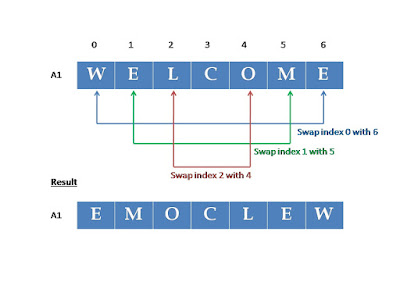`  I request GUEST WRITERS for this web site. Anyone interested please contact me at saravu2k2013@gmail.com Thanks  `
Showing posts with label Data Structures. Show all posts
Showing posts with label Data Structures. Show all posts

Selection sort

Selection sort is an in-place sorting algorithm with the worst case time complexity of O(n2). It works as follows;
Let us consider an array A of size n stored in memory.

• The selection sort algorithm first selects the smallest element in the array A and place it at array index 0; [the index position 0 is sorted now]
• Then it selects the next smallest element in the array A excluding index 0 (considered sorted) and place it at array index 1. [the index positions 0 and 1 are sorted now]
• Then it selects the next smallest element in the array A excluding indexes 0, 1 (considered sorted) and place it at array index 2. [the index positions 0,1, 2  are sorted now]
• The algorithm continues this procedure until it places the biggest element in the last position of the array.

Algorithm/Pseudo-code:

for (i = 1, i n 1; i + +)
{
low_index = i; low_key = A[i];
for (j = i + 1; j n; j + +)
if (A[j] < low_key)
{
low_key = A[j];
low_index = j
}
swap (A[i], A[low_index])
}

Example:

**********

Selection sort recursive program in C in Data structures

Selection sort recursive program in C in Data structures

Program in C:

#include <stdio.h>
#include<conio.h>

int x; //declaration of global variable for array
int selectionSort(int, int); //function declaration

int main()
{
int i, n = 0, total;
printf ("Enter the number of elements you like to sort : ");
scanf("%d", &total);

for (i=0;i<total;i++)
{
printf("Enter the element %d: ", i+1);
scanf("%d", &x[i]);
}

printf (" Array Elements before sorting: ");

for (i=0; i<total; i++)
printf ("%d ", x[i]);

// call selection sort function by passing position and the total number of elements
selectionSort(n, total);

printf ("\n Array Elements after sorting: ");
for (i=0; i<total; i++)
printf ("%d ", x[i]);
getch();
}

int selectionSort( int n, int total)
{
int k, temp, min_key, min_index;
if (n== total-1)
return (-1);
min_key = x[n]; //initially the value at index 0 is considered minimum

min_index = n;

for (k = n+1; k<total; k++)
{
if (x[k] < min_key) //checks whether min_key is minimum or other element. If other element is minimum then the other element value will be stored as new min_key
{
min_key = x[k];
min_index = k;

}
}
temp = x[n]; // swaps x[n] and x[p]

x[n] = x[min_index];
x[min_index] = temp;
n++ ;
selectionSort(n, total); //recursive call of the function

}

***********

In-place algorithm

There are devices that don’t have enough memory space. For example, mobile phones, PDA, etc. For these devices, the problem is more time is spent on I/O (input/output) operation than calculation.

In-place algorithm - an algorithm that uses a small constant amount of extra space in addition to the original input, usually overwrite the input space.

Example:

Reverse the string “WELCOME”.

Method 1:

Assume that the letters are stored in an array A1 as follows;

 W E L C O M E

To reverse the string ‘WELCOME’, we can create another array A2 and read A1 in reverse and paste each element in A2 from the beginning as follows;Non-in-place algorithm uses O(n) extra space

Drawback:
This method needs O(n) additional memory space. That is, we create another array of same size and paste the characters from the given string.

Method 2:

Assume that the letters are stored in an array A1 as follows;

 W E L C O M E

To reverse the string ‘WELCOME’, we can exchange the element stored in the first index with the last, second index with the last but one, and so on until entire string is reversed. You can observe it from the figure below;In-place algorithm - uses O(1) extra space to swap

This method implements in-place algorithm to reverse the given string. O(1) constant extra space is used to reverse the string. [This one character space is used to swap two characters from the string. Temp = A1, A1 = A1, A1 = temp]

Example in-place algorithms:

• Insertion sort
• Selection sort
Example of not-in-place (out-of-place) algorithms:

• Merge sort
******************

in-place sorting algorithm
examples for in-place algorithm
how does in-place algorithm works
how does in-place algorithm save space
how much extra space an in-place algorithm need

SQL exercises for beginners one

SQL Exercises for Beginners / Simple SQL Exercises with Answers / Solved SQL exercises / SQL solved exercises with simple conditions / So...